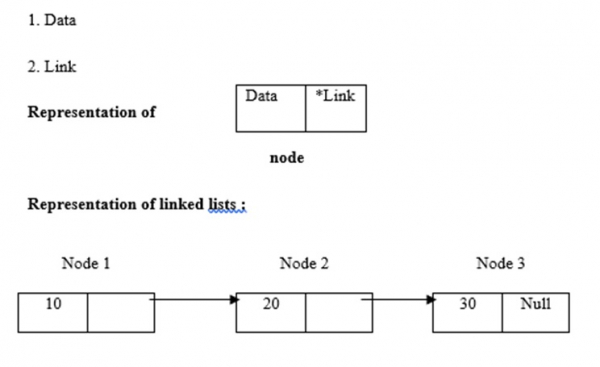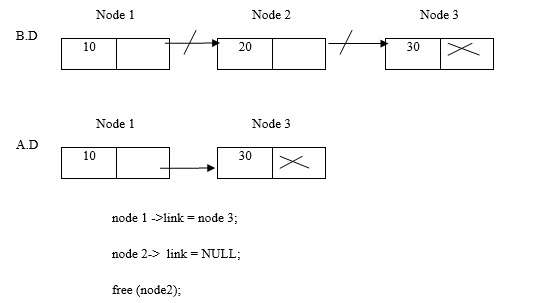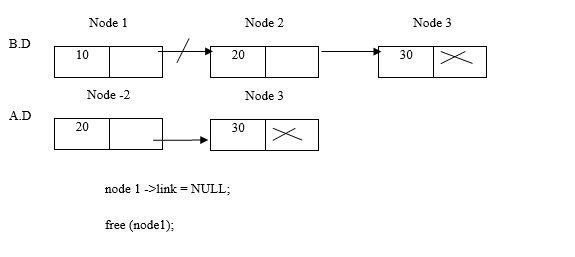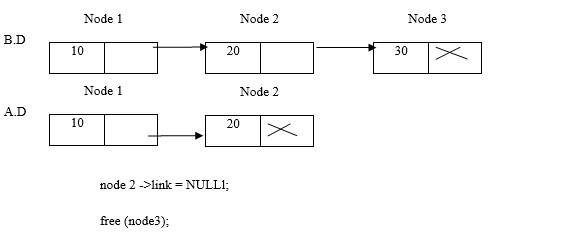# Explain the deletion of element in linked list

CServer Side ProgrammingProgramming

Linked lists use dynamic memory allocation i.e. they grow and shrink accordingly. They are defined as a collection of nodes. Here, nodes have two parts, which are data and link. The representation of data, link and linked lists is given below −There are three types of operations on linked lists in C language, which are as follows −

• Insertion
• Deletion
• Traversing

## Deletion

Consider an example given below −

Delete node 2Delete node 1Delete node 3## Program

Following is the C program for deletion of the elements in linked lists −

Live Demo

#include <stdio.h>
#include <stdlib.h>
struct Node{
int data;
struct Node *next;
};
void push(struct Node** head_ref, int new_data){
struct Node* new_node = (struct Node*) malloc(sizeof(struct Node));
new_node->data = new_data;
}
void deleteNode(struct Node **head_ref, int position){
//if list is empty
return;
if (position == 0){
free(temp);
return;
}
for (int i=0; temp!=NULL && i<position-1; i++)
temp = temp->next;
if (temp == NULL || temp->next == NULL)
return;
struct Node *next = temp->next->next;
free(temp->next); // Free memory
temp->next = next;
}
void printList(struct Node *node){
while (node != NULL){
printf(" %d ", node->data);
node = node->next;
}
}
int main(){
puts("Created List: ");
puts("\n List after Deletion at position 3: ");
}
Created List:
8 2 3 7